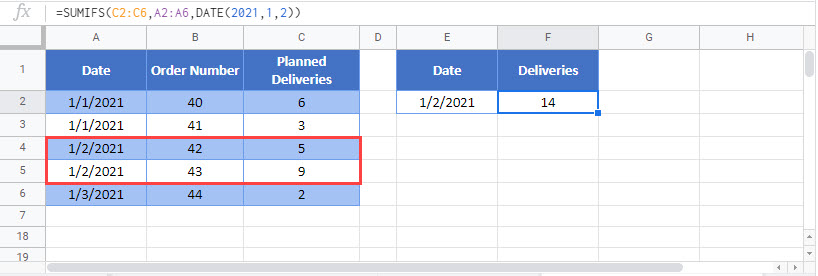# Sum Values if Dates are Equal– Excel & Google Sheets

This tutorial will demonstrate how to use the SUMIFS Function to sum data with specific dates in Excel and Google Sheets.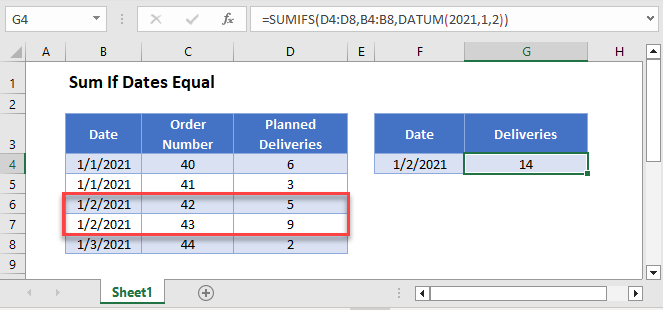## Sum Values if Dates are Equal

The SUMIFS Function sums data that meet certain criteria. Its syntax is: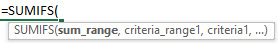This example will sum the number of Planned Deliveries on a specific Date by using the SUMIFS and DATE Functions.

``=SUMIFS(D3:D7,B3:B7,DATE(2021,1,2))``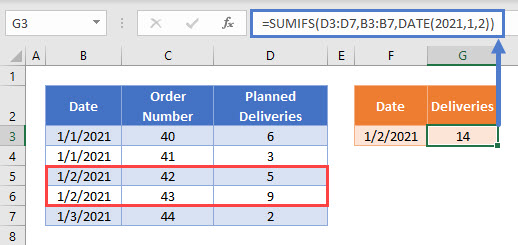In this example, we want to sum Deliveries planned for 1/2/2021. To enter this Date as a criteria in our formula, we use the DATE Function:

``DATE(2021,1,2)``

In this case, the DATE Function expression above is all we need to test whether the date is equal to 1/2/2021. However, a logical test in the SUMIFS Function generally requires an operator in double quotes (“”), so the sum criteria could also be written as:

``"="&DATE(2021,1,2)``

## Sum Values if Dates are Equal – Cell References

Usually, it is bad practice to hard-code date values into formulas. Instead, it is more flexible to use a separate cell to define the date to be used as a sum criteria.

``=SUMIFS(D3:D7,B3:B7,F3)``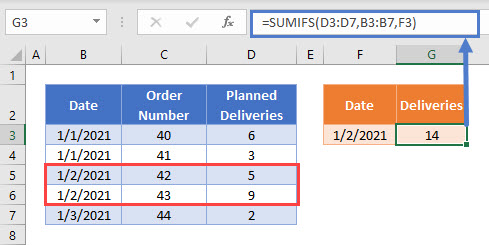This allows the user to change the Date used in the formula in a quicker and more visual way by editing the cell F3.

Note that other logical operators can also be used in SUMIFS, such as:

• Greater than (“>”&F3)
• Greater than or equal to (“>=”&F3)
• Less than (“<“&F3)
• Less than or equal to (“<=”&F3)
• Not equal to (“<>”&F3)

## Locking Cell References

To make our formulas easier to read, we’ve shown the formulas without locked cell references:

``=SUMIFS(D3:D7,B3:B7,F3)``

But these formulas will not work properly when copy and pasted elsewhere in your file. Instead, you should use locked cell references like this:

``=SUMIFS(\$D\$3:\$D\$7,\$B\$3:\$B\$7,F3)``